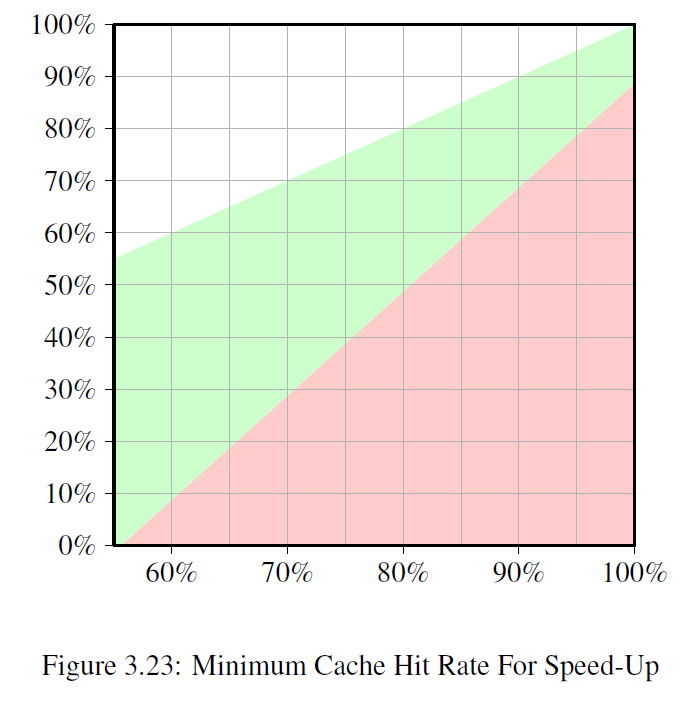# Special Case: Hyper-Threads

Hyper-Threads (sometimes called Symmetric Multi-Threading（对称多线程）, SMT) are implemented by the CPU and are a special case since the individual threads（单个线程） cannot really run concurrently.

They all share almost all the processing resources except for the register set.

ps: 除了寄存器，共享所有的资源。

Individual cores and CPUs still work in parallel but the threads implemented on each core are limited by this restriction（限制）.

## 理论与现实

In theory there can be many threads per core but, so far, Intel’s CPUs at most have two threads per core.

The CPU is responsible（主管，负责） for time-multiplexing（时间多路复用） the threads.

This alone would not make much sense, though.

## 优点

The real advantage is that the CPU can schedule another hyper-thread and take advantage of available resources such as arithmetic logic units（算术逻辑单元） (ALUs) when the currently running hyper-thread is delayed.

In most cases this is a delay caused by memory accesses.

If two threads are running on one hyper-threaded core the program is only more efficient than the single-threaded code if the combined （合计的）runtime of both threads is lower than the runtime of the single-threaded code.

This is possible by overlapping（重叠） the wait times for different memory accesses which usually would happen sequentially.

A simple calculation shows the minimum requirement on the cache hit rate to achieve（实现） a certain speed-up.

## 执行时间的统计

The execution time for a program can be approximated（近似） with a simple model with only one level of cache as follows (see ):

Texe = N * [(1 - Fmem)Tproc + Fmem(Ghit * Tcache + (1 - Ghit)Tmiss)]


The meaning of the variables is as follows:

N = Number of instructions. Fmem = Fraction of N that access memory. Ghit = Fraction of loads that hit the cache. Tproc = Number of cycles per instruction. Tcache = Number of cycles for cache hit. Tmiss = Number of cycles for cache miss. Texe = Execution time for program.

# 双线程执行的效果

For it to make any sense to use two threads the execution time of each of the two threads must be at most half of that of the single-threaded code.

The only variable on either side is the number of cache hits.

If we solve the equation（方程式） for the minimum cache hit rate required to not slow down the thread execution by 50% or more we get the graph in Figure 3.23.## 解释

The input, measured on the X–axis, is the cache hit rate Ghit of the single-thread code.

The Y–axis shows the cache hit rate for the multi-threaded code.

This value can never be higher than the single-threaded hit rate since, otherwise, the single-threaded code would use that improved code, too.

For single-threaded hit rates–in this specific case–below 55% the program can in all cases benefit from using threads.

The CPU is more or less idle（空闲） enough due to cache misses to enable running a second hyper-thread.

### 绿色区域

The green area is the target.

If the slowdown for the thread is less than 50% and the workload of each thread is halved the combined runtime might be less than the single-thread runtime.

For the modeled processor (numbers for a P4 with hyper-threads were used) a program with a hit rate of 60% for the single-threaded code requires a hit rate of at least 10% for the dual-threaded program.

That is usually doable（可行的）.

But if the single-threaded code has a hit rate of 95% then the multi-threaded code needs a hit rate of at least 80%.

That is harder.

Especially, and this is the problem with hyper-threads, because now the effective cache size (L1d here, in practice also L2 and so on) available to each hyper-thread is cut in half.

Both hyper-threads use the same cache to load their data.

If the working set of the two threads is nonoverlapping（不重叠） the original 95% hit rate could also be cut in half and is therefore much lower than the required 80%.

• 直接减半的个人理解

## 超线程的适用场景

Hyper-threads are therefore only useful in a limited range of situations.

The cache hit rate of the single-threaded code must be low enough that given the equations above and reduced cache size the new hit rate still meets the goal.

Then and only then can it make any sense at all to use hyper-threads.

Whether the result is faster in practice depends on whether the processor is sufficiently able to overlap（交叠） the wait times in one thread with execution times in the other threads.

The overhead of parallelizing the code must be added to the new total runtime and this additional cost often cannot be neglected（被忽视的）.

In section 6.3.4 we will see a technique where threads collaborate（合作） closely and the tight coupling（紧耦合） through the common cache is actually an advantage.

This technique can be applicable to many situations if only the programmers are willing to put in the time and energy to extend their code.

What should be clear is that if the two hyper-threads execute completely different code (i.e., the two threads are treated like separate processors by the OS to execute separate processes) the cache size is indeed cut in half which means a significant（重大） increase in cache misses.

Such OS scheduling practices are questionable unless the caches are sufficiently large.

Unless the workload for the machine consists of processes which, through their design, can indeed benefit from hyper-threads it might be best to turn off hyper-threads in the computer’s BIOS.

p29